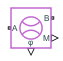# Flow Rate Sensor (G)

Measure mass, energy, and volumetric flow rates in gas networks

Since R2023a

•Libraries:
Simscape / Foundation Library / Gas / Sensors

## Description

The Flow Rate Sensor (G) block represents an ideal sensor that measures mass flow rate, energy flow rate, and volumetric flow rate in a gas network. The energy flow rate is the advection of total enthalpy. There is no change in pressure or temperature across the sensor.

Because the flow rates are Through variables, the block must connect in series with the component being measured.

The relative orientation of ports A and B establishes the measurement sign. The sign is positive if flow occurs from port A to port B. Switching port connections reverses the measurement sign.

Three physical signal ports are available to output the measurement data:

• Port M outputs the mass flow rate.

• Port V outputs the volumetric flow rate.

• Port φ outputs the energy flow rate.

These ports have conditional port visibility on the block icon. Use the block parameters to expose only the ports that you need for measurements in a particular model.

Connect the measurement ports to PS-Simulink Converter blocks to transform the output physical signals into Simulink® signals, for example, for plotting or additional data processing.

## Ports

### Output

expand all

Physical signal output port for mass flow rate measurement.

#### Dependencies

To enable this port, select the Mass flow rate check box.

Physical signal output port for the volumetric flow rate measurement.

#### Dependencies

To enable this port, select the Volumetric flow rate check box.

Physical signal output port for energy flow rate measurement.

#### Dependencies

To enable this port, select the Energy flow rate check box.

### Conserving

expand all

Gas conserving port. Flow rates are positive if gas flows from port A to port B.

Gas conserving port. Flow rates are positive if gas flows from port A to port B.

## Parameters

expand all

Selecting this check box exposes the output port M, which lets you measure mass flow rate.

Selecting this check box exposes the output port V, which lets you measure volumetric flow rate.

Select whether the sensor calculates volumetric flow rate at actual working conditions or at a standard pressure and temperature:

• `Actual conditions` — The sensor calculates the volumetric flow rate by using density at the actual working conditions of the system.

• `Standard conditions` — The sensor calculates the volumetric flow rate by using density at standard conditions. When you select this option, additional parameters become available to let you specify the standard pressure and temperature.

#### Dependencies

To enable this parameter, select the Volumetric flow rate check box.

Gas pressure at standard conditions.

#### Dependencies

To enable this parameter, set Volumetric flow measurement conditions to `Standard conditions`.

Gas temperature at standard conditions.

#### Dependencies

To enable this parameter, set Volumetric flow measurement conditions to `Standard conditions`.

Selecting this check box exposes the output port φ, which lets you measure energy flow rate.

## Version History

Introduced in R2023a

expand all